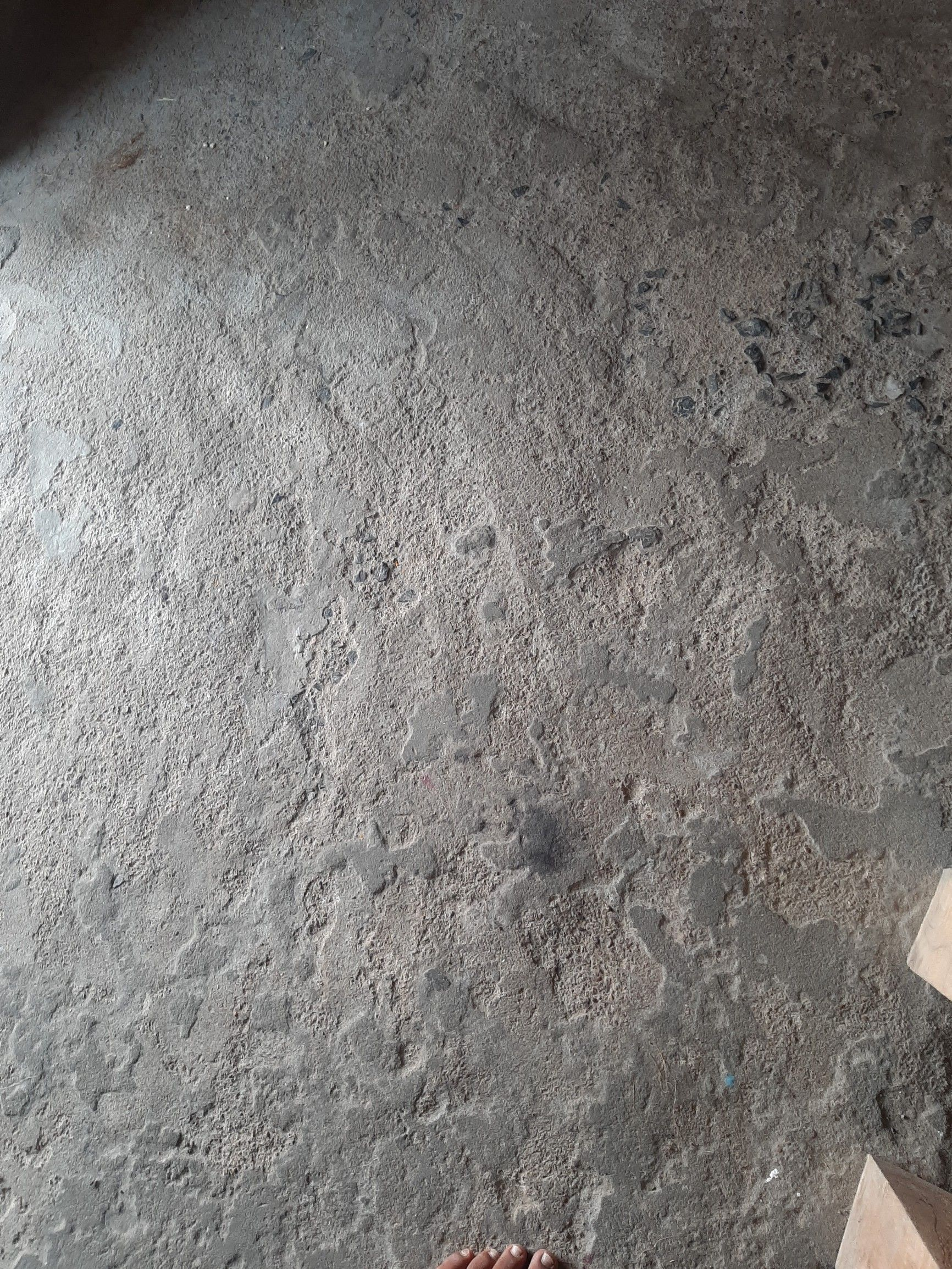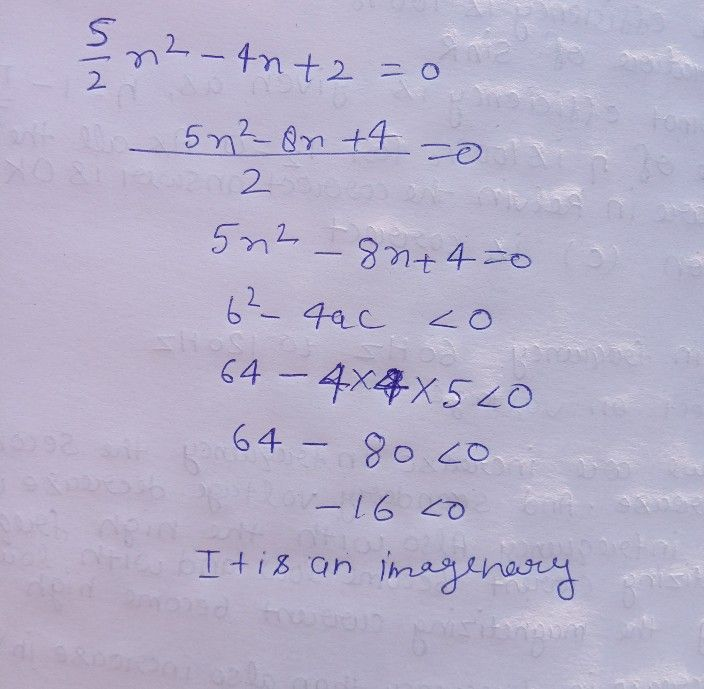Symbol
Problem$5$ $\dfrac {5} {2}x^{2}-4x+2=0$
7th-9th grade
Other
Question content
Characterize the nature of the roots of the quadratic equation using discriminant.
SolutionQanda teacher - Atulthakur